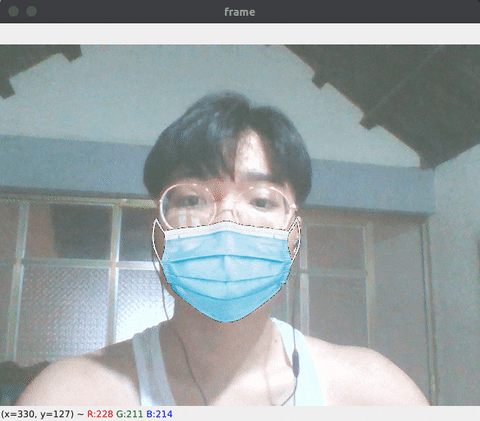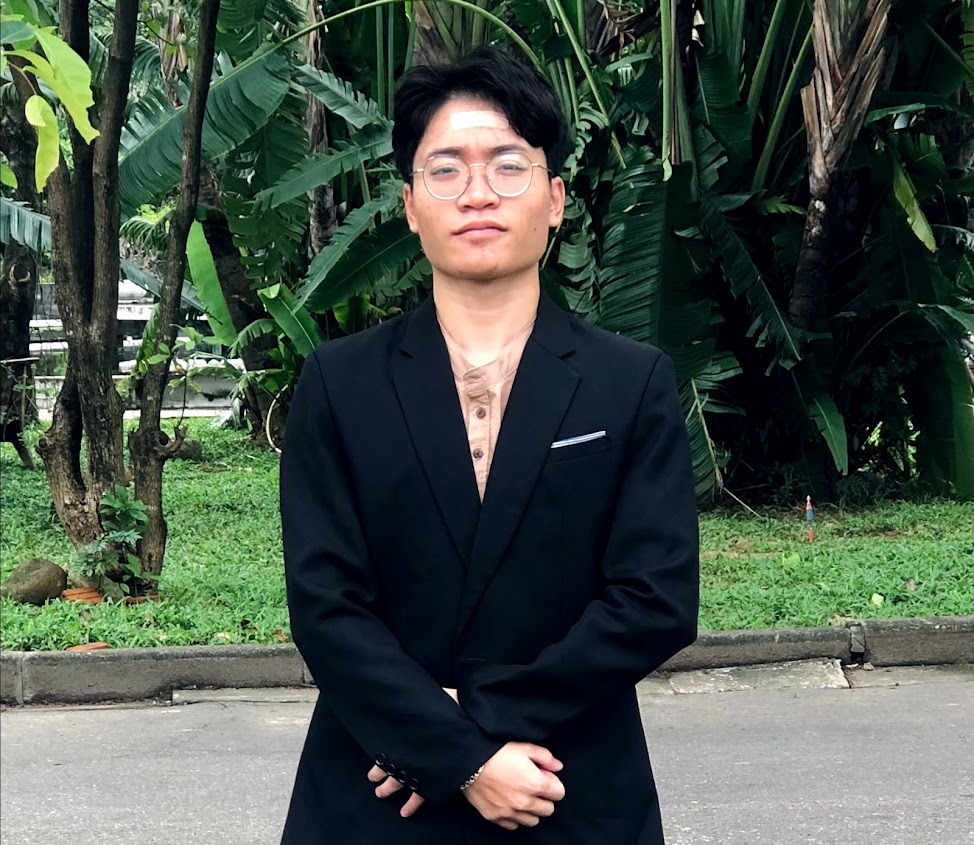Kudo Khang — Published On August 4, 2021

This article was published as a part of the Data Science Blogathon

## Introduction

Computer vision is an increasingly popular keyword, its applications appear everywhere in life from solving work to entertainment. In this article, I would like to introduce to you some common techniques commonly used in computer vision and image processing: Bitwise operator, slicing technical. And then, creating an interesting application: Wearing mask from video.

• Bitwise Operator in OpenCV, Slicing Technical.
• A challenge for you.

## Bitwise operator

Bitwise operations are performed on an image when we need to extract only the required parts of the image. When we need to extract an irregular-shaped object from one image and paste it on another image. That’s exactly when we use bitwise operations on the image to separate the foreground from the background.

In computer programming, bitwise operations operate on one or more decimal numbers. This is a simple and fast math operation that is directly supported by the processor. There are a total of 7 bitwise operations, but in this article, we only need to learn 3 operators AND, OR & NOT.

 Name Description AND If both bit is 1, return value is 1, otherwise, return 0. OR If either bit is 1, the return value is 1, otherwise 0. NOT Inverts all bits, 0 to 1 and 1 to 0.

OpenCV allows us to implement these 3 operators directly: `cv2.bitwise_and`, `cv2.bitwise_or`,  `cv2.bitwise_not`

```import cv2
# bitwise by opencv
bitwise_AND = cv2.bitwise_and(circle, square)
bitwise_OR = cv2.bitwise_or(circle, square)
bitwise_NOT = cv2.bitwise_not(circle)
# show results
cv2.imshow('circle', circle)
cv2.imshow('square', square)
cv2.imshow('AND', bitwise_AND)
cv2.imshow('OR', bitwise_OR)
cv2.imshow('NOT', bitwise_NOT)
# press 'q' to quit
if cv2.waitKey(0) & 0xFF == ord('q'):
cv2.destroyAllWindows()```

And here is the result:AND, OR & NOT

As you know, in the binary image, white is 1 and black is 0.

Take a look at the AND operator table below and compare it with the image results `cv2.bitwise_and`:

 Operator Result 0 AND 0 0 0 AND 1 0 1 AND 0 0 1 AND 1 1

We can see that the results are very consistent, right? Black and black produces black, white and black, black and white produces the same result. Only white and white areas will produce white results.

The same goes for the OR operator:

 Operator Result 0 OR 0 0 0 OR 1 1 1 OR 0 1 1 OR 1 1

Only the black OR black produces black, the rest all produce white.

And finally the NOT operator. It inverts all bits 0 to 1 and 1 to 0. Very easy to understand, right.

 Operator Result NOT 0 1 NOT 1 0

## Create a medical mask filter

Now we will create an interesting project.

Idea: You must have used or known filters when video calling on Facebook, for example adding rabbit ears, dog tongue, pig nose… In this project we will write a similar program, wearing a mask for face from the webcam.Result

Step by step:

1. Install necessary libraries.
2. Face recognition, identify mouth area.
3. Use the masking technique to get the true area of the medical mask.
4. Carry out mask grafting to the identified mouth area.

## 1. Install libraries.

Implementation environment:

• Ubuntu 20.04
• Python 3.9
``````pip install opencv-python
``````pip install dlib
````pip install numpy````

You just need to download, the details for each library will be explained in the following section. Create `main.py` and import the library:

``````import cv2
``````import numpy as np
``````import dlib
````from math import hypot````

Note: The `math` library is available so we don’t need to install it anymore.

## 2. Detecting mouth area on the face

There are several ways to recognize faces, for the easiest in this article, I will use the Dlib library (shape_predictor_68_face_landmarks.dat) to recognize faces and get landmarks for calculating the mouth area.

Facial landmarks:68 facial landmarks

To use Dlib library we initialize as follows:

``````detector = dlib.get_frontal_face_detector()
predictor = dlib.shape_predictor("shape_predictor_68_face_landmarks.dat")``````

``````cap = cv2.VideoCapture(0) # if you using camera, change 0 to 1

We need to put the code in a loop to get the landmarks:

``````while True:
``````    _, frame = cap.read()
``````    frame = cv2.flip(frame, 1)
``````    frame_gray = cv2.cvtColor(frame, cv2.COLOR_BGR2GRAY)
``````    faces = detector(frame)
``````    for face in faces:
````        landmarks = predictor(frame_gray, face)````

There will be 68 landmarks recognized and returned as a tuple(x,y).
You can check by `print(landmarks.part(num)).`
`(num` is a number from 0 to 67)

Next, we need to define a rectangle as the mouth area to add the mask to:Define a rectangle as the mouth area

But before that, we will take out 3 special points to serve the calculation:

``````bottom_mask = (landmarks.part(9).x, landmarks.part(9).y)
top_left = (landmarks.part(1).x, landmarks.part(1).y)
top_right = (landmarks.part(16).x, landmarks.part(16).y)``````

We have to calculate its width and height. The width will be the space between `top_left` and `top_right`. The formula for `width` and `height` would be:

`w/h` is the width/height ratio of the medical mask, such a calculation helps to keep the correct proportions when merging into the image.

In Python, we can easily calculate the distance between two points using the `hypot` function:

``````mask_width = int(hypot(top_right - top_left, top_right - top_left))
````mask_height = int(mask_width * w/h)````

## 3. Extract the medical mask area

Resize the medical mask image to the exact size of the rectangle we just calculated:

``mask = cv2.resize(mask_img, (mask_width, mask_height))``

The image is now an RGB image, ie 3-dimensional (width, height, channel=3) we need to convert it to a grayscale image to get a 2-dimensional image (width, height, channel=1) for convenience to calculate.

``mask_gray = cv2.cvtColor(mask, cv2.COLOR_BGRA2GRAY)``

Create a binary mask to get the correct part of the medical mask:

``_, mask_mask = cv2.threshold(mask_gray, 25,255, cv2.THRESH_BINARY_INV)``(You can see more about the binary technique `cv2.threshold()` here)

Next, we need to cut the rectangle of the mouth area that we mentioned in the previous step. But in the beginning, let’s take a look at the 2-D array Slicing technique.

(You can skip this step if you already understand it)

Initialize a 2D array:

``arr = np.array(np.arange(100).reshape(10, 10))``

We will get a 2D array like this:Initialize a 2D array

Supposed to cut a rectangle with (width=4 and height=5) highlighted in green, how do we do that? The slicing technique will help us do just that:

`rec = arr[3:3+5, 3:3+4]`Slicing array 2D

Then, try to change all values of `rec` to zero and reassign `rec` to `arr`:

``````rec = np.zeros((5,4))
arr[3:3+5, 3:3+4] = rec``````

The `arr` array will now be:Array after processing

Make sure you understand the slicing technique I just demonstrated because that’s exactly what we’re going to do next.

Slicing the mouth area:

``area = frame[top_left : top_left + mask_height , top_left : top_left + mask_width]``

Create mouth area without medical mask using `cv2.bitwise_and()`

`area_no_mask = cv2.bitwise_and(area, area, mask = mask_mask)`
``

Mouth area without the medical mask

Finally, use `cv2.add()` to concatenate `area_no_mask` and `mask`:

``final = cv2.add(area_no_mask, mask)``

You will be:Final

The last thing we just need to do is return the processed `area` area to `frame`:

``frame[top_left - weight : top_left + mask_height - weight, top_left : top_left + mask_width] = final``

Display the final result by `cv2.imshow()`:

``````cv2.imshow('Result', frame)
# press 'q' to break
if cv2.waitKey(0) & 0xFF == ord('q'):
break``````And here is the result :3

## Summary

Through this article, I have presented masking, slicing technical and bitwise operators. Those are important and commonly used techniques in image processing.

This recognition technology is still limited, using `Dlib` it can only recognize 68 landmarks so the accuracy is not high, you can try with Google’s `Mediapipe` (with 468 landmarks) to try to improve your program.

## Challenge

To make sure you have a good understanding of what’s covered, write a program similar to the following:

Idea: Add a pig’s nose to the face from a webcam
Perform: Calculate nose part from webcam, perform pig nose grafting. The steps are the same as above.

Thank you for reading my article, all the source codes of these 2 projects can be downloaded here. Connect with me on Linkedin if you have any questions.